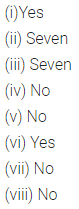ML Aggarwal Class 6 Solutions Chapter 10 Basic Geometrical Concept Check Your Progress for ICSE Understanding Mathematics acts as the best resource during your learning and helps you score well in your exams.

## ML Aggarwal Class 6 Solutions for ICSE Maths Chapter 10 Basic Geometrical Concept Check Your Progress

Question 1.
(i) Name all the rays shown in the given figure whose initial point is A.
(ii) Is ray $$\overrightarrow{\mathrm{AB}}$$ different from ray $$\overrightarrow{\mathrm{AD}}$$ ?
(iii) Is ray $$\overrightarrow{\mathrm{CA}}$$ different from ray $$\overrightarrow{\mathrm{CE}}$$ ?
(iv) Is ray $$\overrightarrow{\mathrm{BA}}$$ different from ray $$\overrightarrow{\mathrm{CA}}$$ ?
(v) Is ray $$\overrightarrow{\mathrm{ED}}$$ different from ray $$\overrightarrow{\mathrm{DE}}$$ ?Solution: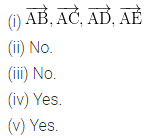Question 2.
From the given figure, write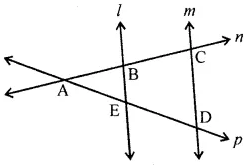(i) all pairs of parallel lines.
(ii) all pairs of intersecting lines.
(iii) lines whose point of intersecting is E.
(iv) collinear points.
Solution: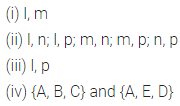Question 3.
In the given figure :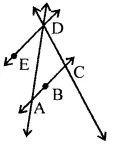(a) Name;
(i) Parallel lines.
(ii) All pairs of intersecting lines.
(iii) concurrent lines.
(b) State wheather true or false:
(i) points A, B and D are collinear.
(ii) lines AB and ED interesect at C.
Solution:Question 4.
In context of the given figure, state whether the following statements are true (T) or false (F):(i) Point A is in the interior of ∠AOD.
(ii) Point B is in the interior of ∠AOC.
(iii) Point C is in the exterior of ∠AOB.
(iv) Point D is in the exterior of ∠AOC.
Solution: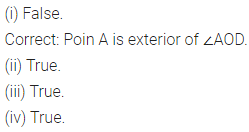Question 5.
How many angles are marked in the given figure? Name them?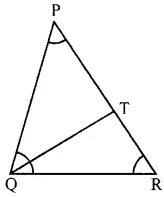Solution: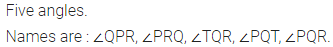Question 6.
In context of the given figure, name
(i) all triangles
(ii) all triangles having point E as common vertex.Solution: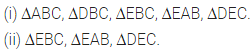Question 7.
In context of the given figure, answer the following questions: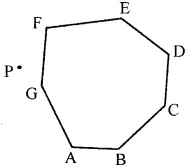(i) Is ABCDEFG a polygon?
(ii) How many sides does it have?
(iii) How many vertices does it have?
(iv) Are $$\overline{\mathrm{AB}}$$
and $$\overline{\mathrm{FE}}$$ adjacent sides?
(v) Is $$\overline{\mathrm{GF}}$$
a diagonal of the polygon?
(vi) Are $$\overline{\mathrm{AC}}, \overline{\mathrm{AD}} \text { and } \overline{\mathrm{AE}}$$ diagonals of the polygon?
(vii) Is point P in the interior of the polygon?
(viii)Is point A in the exterior of the polygon?
Solution: